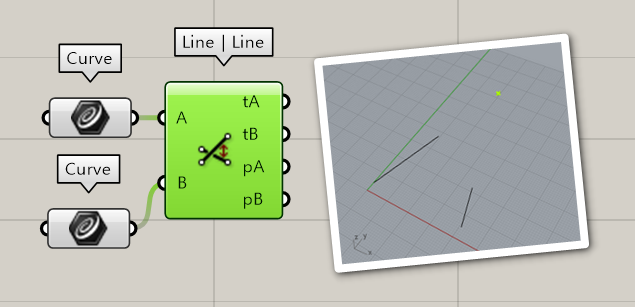# Lines, Line segments and vectors

Hello guys, this is both a theoretical and a practical question:

In geometry, you have

• Lines: “A line is a straight one-dimensional figure having no thickness and extending infinitely in both directions.”
• Line segments: “the part of a line between two points A and B”
• Vectors: “a quantity having direction as well as magnitude”

In Grasshopper, so far I’ve found line segments (called lines), vectors, but I haven’t found -mathematical- lines.
to give you a practical example, I am trying to find the intersection between two lines which in their turn are intersections between planes. The only way I have found so far is to extend them by an arbitrary amount on both ends so as to be sure they will meet.

Isn’t there an entity like the mathematical line that extends infinitely?
(both examples in the picture are the same components with the same input)

Looking for “LLX”?I guess so, (I was expecting something that only works if they are coplanar)
this component essentially gives you the distance between the lines.
for all practical purposes though, this more than does the work! -after all you can do a planarity check, or make sure the distance between the points is zero.
Thanks Kim! I consider my question -practically- answered!

In mathematics I believe the preferred nomenclature is:

• Line (infinite in both directions)
• Ray (infinite in one direction, it has a start, but no end)
• Segment (finite length)

In Rhino we use “Line” for both lines and segment, although we do use “Ray”. Whether or not a line is taken to be finite or infinite in Rhino is contextual. We do use “Segment” when talking about the pieces that make up a polyline.

In everyday language “line” is often used when mathematicians or programmers would say “curve” or “spline”.

In Grasshopper, if the parameter is of type Curve and you feed it a line, it will automatically be converted into a finite line segment. Only components that take in Line data specifically can choose whether or not to use infinite lines.

3 Likes

absolutely clear! thank you.